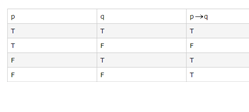# Truth Tables for Conditionals Worksheets

What Are the Trends of Truth Tables for Conditionals? The basics of mathematical logic goes with the if and is conditional statements. A conditional statement, symbolized by p → q, is an if-then statement in which p is a hypothesis and q is a conclusion. The logical connector in a conditional statement is denoted by the symbol →. The conditional is defined to be true unless a true hypothesis leads to a false conclusion. The table for truth statements goes as follows:In the truth table above, p → q is only false when the hypothesis (p) is true, and the conclusion (q) is false; otherwise, it is true. It means there are more chances for a statement to be true then to be false. If a true hypothesis leads to a false conclusion, then the conditional statement is considered true. Note: A conditional statement is a compound statement.

• ### Basic Lesson

Demonstrates the concept of determining truth values for Conditionals.

• ### Intermediate Lesson

Shows students how to determine truth values for Conditionals. Use a truth table to determine the possible truth values of the given statement.

• ### Independent Practice 1

Contains a mixture of problems using Conditionals. Students must determine the truth value. Using the tables below, determine the truth values of the following statements.

• ### Independent Practice 2

Features truth value questions with assorted concepts. Students concentrate on Conditionals.

• ### Homework Worksheet

Features 6 conditional problems; students must determine the truth-value.

• ### Skill Quiz

10 truth value questions that include Conditionals. Scoring matrix is provided.

• ### Homework and Quiz Answer Key

Answers for the homework and quiz.